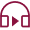Mutual Funds
Last Updated: 2022-10-19T14:12:36+05:30## Introduction

Earning returns is the primary goal of any investment. A return can take the form of income, capital appreciation, or both. Compound annual growth rate (CAGR) and Extended Internal Rate of Return (XIRR) are mutual funds' most popular return measures.

An investment's CAGR represents its annual growth rate assuming compounding every year. The CAGR, however, does not apply to multiple cash flows; it applies to XIRR in mutual funds. But what is XIRR in MF?

## What is XIRR in Mutual Funds?

As mentioned above, XIRR stands for Extended Internal Rate of Return. An Extended Internal Rate of Return (XIRR) calculates the total current value of an investment by applying a single rate of return to each instalment (or redemption).

An investor's return rate is their XIRR. In other words, it is the actual return on your investment.

It can be a bit complicated to calculate returns considering the multiple investments (and therefore multiple purchase prices) and different periods for each instalment in a SIP. Hence, a mutual fund SIP's return is typically calculated using  XIRR.

## Why Does it Make Sense for Mutual Fund Investments?

Let’s understand XIRR's meaning in mutual funds in detail.

Investors can invest a lump sum amount in a mutual fund with two cash flows. This means they will only have one cash outflow (from investments) and one cash inflow (from redemptions). The CAGR method (which reflects a mutual fund's growth rate per year over an investment period) is the most appropriate in such cases.

However, investors who invest in mutual fund schemes through Systematic Investment Plans (SIP) or redeem units of mutual fund investment through Systematic Withdrawal Plans (SWP) cannot take advantage of this. CAGR cannot be used to calculate returns from a mutual fund because of the multiple cash flows involved. Therefore, using XIRR makes sense.

To understand XIRR, one must first understand IRR.

## What Is an IRR?

An IRR (Internal Rate of Return) measures the return on a series of cash flows. The Investor Return Ratio (IRR) estimates returns on investments, such as SIPs, SWPs, lump-sum investments with additional purchases, multiple redemptions, etc. IRR, however, has some limitations.

IRR formulas in Excel automatically assume that cash-flow intervals are the same throughout the tenure of the investment, which rarely occurs.

Take a look at an example!

An investor makes 53,000 after investing  5,000, 4,000, 4,000, 6,500, and 9,000 in SIPs during the tenure. Based on the IRR calculation method, the return on investment is 22%.

However, investments in mutual funds are not so regular (even if they are enrolled in a systematic investment plan) since there are holidays, fewer days in some months, etc. At various points in time, there will be cash inflows and outflows. Aside from the amount invested, the duration of the investment also has an impact on the outcome.

Hence, XIRR can determine the right return on multi-cash flow mutual funds investments.

## XIRR Calculation

Now that you’re familiar with XIRR meaning, let’s look at its calculations.

Applying the XIRR formula to Mutual Funds in Excel and following the steps below will calculate your XIRR.

1.  Make a column for all your investment transactions, marking outflows as negatives like investments and acquisitions and inflows as positives like redemptions.

2.  In the next column, enter the investment date.

3.  The next column should include your investments' current value and date.

4.  Use the XIRR function in Excel, which looks like =XIRR (values, date, Guess).

5.    Finally, select cash flow values corresponding to the date column in the payment schedule. Include the investment dates in the date column along with the cash flow dates. The Guess parameter is optional, and if no value is entered, Excel defaults to 0.1.

## Example of How to Use the XIRR Function in Excel

Let's take a look at how this formula works in Excel.

Suppose we have a set of cash flows like those in the table below:

 Date Cash flows (in Rs) 01 January 2016 -50,000 10 January 2016 500 01 June 2016 500 25 October 2016 500 27 October 2016 500 01 March 2017 500 15 June 2017 51,000 XIRR 4.89%

Interest inflows are irregular in the table above. Hence, to calculate the return on these cash flows, you can use the XIRR function.

## Open an Excel Sheet and Follow these Steps:

●    Enter the original investment amount in an Excel sheet. Use A 'minus' sign to represent the amount invested.
●    Enter each period's returns in the following cells. Every time you invest money, remember to include the minus sign.
●    Now, find XIRR using the function: “=XIRR(values, dates, guess)”

The term values refer to a series of cash flows corresponding to a schedule of payments. During the investment period, the first payment represents the investment made at the beginning. Afterwards, all payments are discounted according to a 365-day calendar. The series of values must have at least one positive and one negative value.

The date represents the day that the first investment was made, as well as when the first returns were received. The above table shows each date corresponds to the investment made or income received. Dates should be entered in the DD-MM-YY format (date-month-year) to avoid errors. Any invalid date number, or an inconsistent date format, will result in the “#VALUE!” error.

## Conclusion

Both XIRR and CAGR are used to calculate mutual fund returns. When it comes to single payment contributions, CAGR is the most commonly employed formula, while when it comes to SIP instalments, XIRR is used most commonly. For investors, it's usually better to know how return calculations work so that they aren't reliant on others.

Open Free Demat Account
Resend OTP